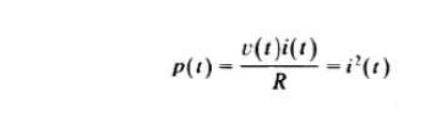Home | | Signals and Systems | Classification of CT and DT signals

# Classification of CT and DT signals

Periodic and non-periodic Signals, Deterministic and Random Signals, Energy and Power Signals:, CT Systems and DT Systems,

Classification of CT and DT signals:

Periodic and non-periodic Signals

A periodic function is one which has been repeating an exact pattern for an infinite period of time and will continue to repeat that exact pattern for aninfinite time. That is, a periodic function x(t) is one for which

x (t) = x(t+nT)

for any integer value of n, where T >0 is the period of the function and − ∞ < t <∞ . The signal repeats itself every T sec. Of course, it also repeats every 2 T,3T and nT. Therefore, 2T, 3T and nT are all periods of the function because the function repeats over any of those intervals. The minimum positive interval over which a function repeats itself is called the fundamental period T0.T0 is the smallest value that satisfies the condition x ( t ) = x ( t+T0). The fundamental frequency f 0 of a periodic function is the reciprocal of the fundamental period f0=1/T0. It is measured in Hertz and is the number of cycles (periods) per second. The fundamental angular frequency ω0 measured in radians per second is ω0=2πT0= 2πf0. A signal that does not satisfy the condition in (2.1) is said to be a periodic or non-periodic.

Deterministic and Random Signals

Deterministic Signals are signals who are completely defined for any instant of time, there is no uncertainty with respect to their value at any point of time. They can also be described mathematically, at least approximately. Let a function be defined asA random signal is one whose values cannot be predicted exactly and cannot be described by any exact mathematical function, they can be approximately described.

Energy and Power Signals:

Consider v(t) to be the voltage across  a resistor R producing a current i(t). The instantaneous power p(t) per ohm is defined as, Total energy E and average power P on a per-ohm basis areFor an arbitrary continuous-time signal x (t), the normalized energy content E of x(t) is defined as,The normalized average power P of x (t) is defined as,Similarly, for a discrete-time signal x[n], the normalized energy content E of x[n] is defined as,The normalized average power P of x[n] is defined as,CT Systems and DT Systems:

A system is defined as a physical device which contains set of elements or functional blocks and that generates a response or output signal for a given input.

Study Material, Lecturing Notes, Assignment, Reference, Wiki description explanation, brief detail
Signals and Systems : Classification of Signals and Systems : Classification of CT and DT signals |